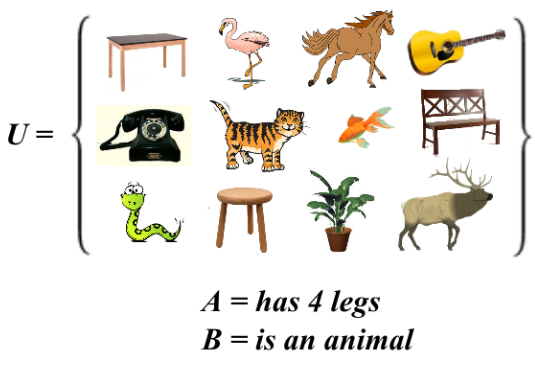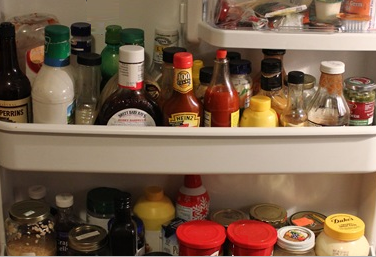Probability

# Set Operations: Level 1 ChallengesWhat is $|A^{C}\cup B^{C}|$?

Details:

$|S|$ represents the cardinality of the set $S$ and $S^C$ represents the complement of the set $S$.

True or False?

$\left|X^C \cup Y^C \cup Z^C\right| = \left|(X \cap Y \cap Z)^C\right|$

True or false:

$\quad$ There exist three nonempty sets $A$, $B$, and $C$ such that
$\quad$ $A\cup (B-C)\neq (A\cup B)-(A\cup C)$.In my fridge's shelves there are 10 condiments that are good for breakfasts, 8 that are good for lunch/dinner, and 12 that are good for dessert.

Of all of the condiments, exactly half of those that are good for breakfast are also good for lunch/dinner. And every condiment is either good for desert or good for both lunch/dinner and breakfast.

Given all of this, what is the difference between the maximum number of condiments that I might have in my fridge and minimum number of condiments that I might have in my fridge?

If $A$ and $B$ are subsets of $U=\{1, 2, 3, 4, 5, 6, 7, 8, 9\}$ such that ${ A }^{ c }\cap { B }^{ { c } }=\{1, 9\}, A\cap B =\{2\}, { A }^{ c }\cap B=\{4, 6, 8\},$ what is the set $A?$

×